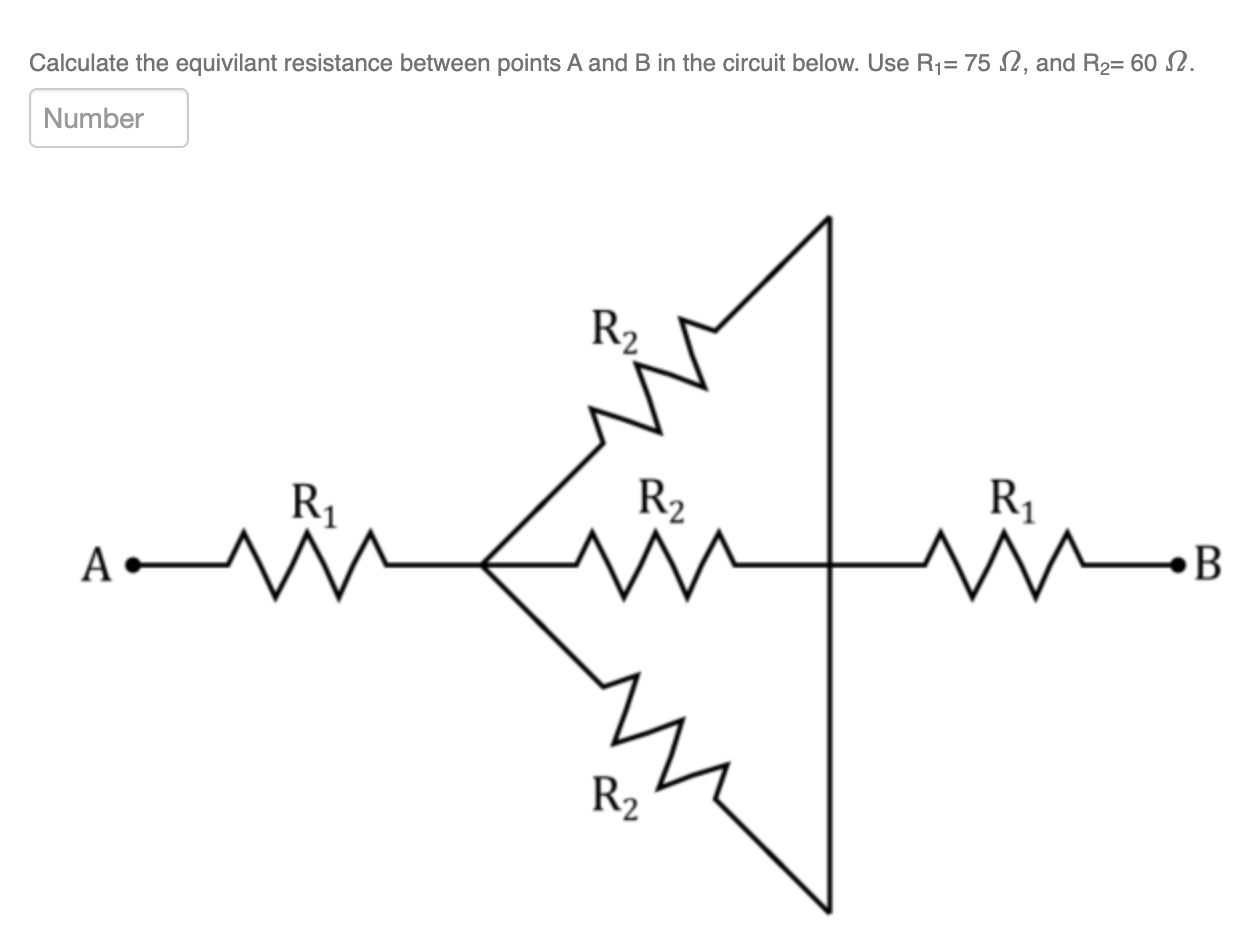# Question Calculate the equivilant resistance between points A and B in the circuit below. Use R1= 75 12, and R2= 60 12. Number R2 R1 R2 A W AC A M B R2Transcribed Image Text: Calculate the equivilant resistance between points A and B in the circuit below. Use R1= 75 12, and R2= 60 12. Number R2 R1 R2 A W AC A M B R2
More
Transcribed Image Text: Calculate the equivilant resistance between points A and B in the circuit below. Use R1= 75 12, and R2= 60 12. Number R2 R1 R2 A W AC A M B R2